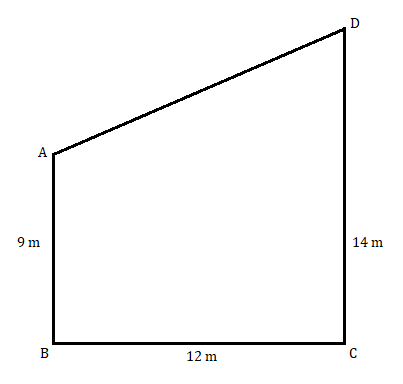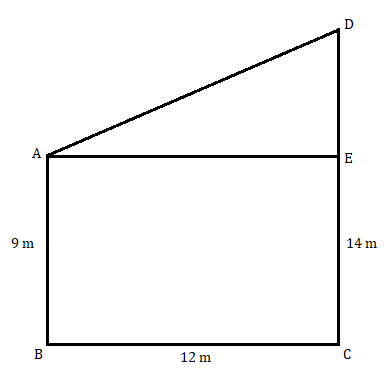# Two vertical poles of height 9 m and 14 m stand on a plane ground. If the distance between their feet is 12 m, find the distance between their tops.

Given: Two vertical poles of height 9 m and 14 m stand on a plane ground and the distance between their feet is 12 m.

To find: We have to find the distance between the tops of the tower

Solution:

In the below diagram, AB is the 9 m pole, DC is the 14 m pole and BC is the distance between their feet.To find the value of AD first draw a line from point A to point E on side DC parallel to BC.Now,

EC = AB = 9 m

AE = BC = 12 m

In ∆AED apply Pythagoras theorem;

So, the distance between their tops is 15 m.

Updated on: 10-Oct-2022

41 Views